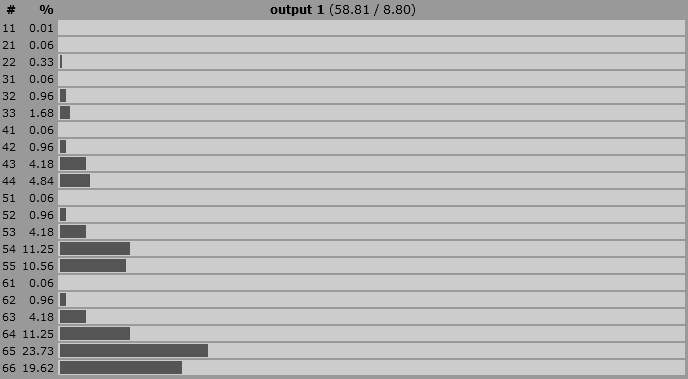# Function to calculate "Nd6 take highest 2, sort descending" probabilities in AnyDice

I'm creating an injury-table where the player has to roll a d66 to see how severe the injury her character sustains is.

The players roll up to 5d6 (they roll more dice if the injury is worse), take the highest two, whereas the higher of the two is the tens digit and the other one is the ones digit. For example, if I roll: 5, 3, 2, 6, I pick the highest two, 5, 6; the higher one goes first, so the result is 65. So the possible values are: 1, 2, 3, 4, 5, 6, 11, 21, 22, 31, 32, 33, 41, 42, 43, 44, 51, 52, 53, 54, 55, 61, 62, 63, 64, 65, 66

In the table, 66 is of course worse for the player than a 1. I need to figure out however, how likely it is to roll for example 1-21, or 22-33 so I can assign the injuries' severity to those numbers. I'm fine as a first step with a function that only considers 2d6.

• Are you asking for a method to roll a d66 (i.e. with equal probability for each number) or for what the distribution of your described method is? (I ask, because what you describe is not a d66.) Nov 2 '19 at 23:34
• I basically need the probability of i.e. rolling a 66 compared to a 21 using two dice. And is it equal probability? A 15 can never occur, only a 51. So this throws me off a bit. @Someone_Evil Sorry, I know it's technically not d66, what else should I call it? Nov 2 '19 at 23:36
• It's probably better to just refer to it as a special roll, rather than d66 or d66-like because it will be less confusing that way Nov 3 '19 at 0:02
• If the severity of the wounds don't depend on the full details of the number (only what range it falls in), you may just want to examine the largest die and use it as your category without regard to the second value. That's a lot easier to program and think about! Nov 3 '19 at 0:21
• Welcome to RPG.SE! Take the tour if you haven't already, and check out the help center for more guidance.
– V2Blast
Nov 3 '19 at 0:40

You can use a function to do this, like so:

function: injury ROLL:s {
result: 1@ROLL * 10 + 2@ROLL
}

output [injury 5d6]


You provide the function a dice pool (such as 5d6). The function casts that pool to a sequence (:s), which means it calculate the result for every possible permutation of the dice pool. When dice pools are cast to sequences like this, the resulting sequence is sorted in descending order by default so we know the 1st die (1@ROLL) will be the tens digit and the 2nd die (2@ROLL) will be the ones digit - we multiply and add accordingly to get your final result.

The output for 5d6, for instance, looks like this:• I will accept this answer because you also provided a detailed description of how this function works! Nov 2 '19 at 23:49
function: highest DIGITS:n of ROLL:s in base BASE:n {
SUM: 0
loop I over {1..DIGITS} {
SUM: BASE * SUM + I@ROLL
}
result: SUM
}

output [highest 2 of 5d6 in base 10]


This is a slightly more general solution that lets you extract any number of the highest* rolled numbers as digits in any base.

Like Carcer's answer, this solution also works by passing the 5d6 roll to a function expecting a sequence. This makes AnyDice call the function for every possible (sorted) outcome of the roll and collect the results into a custom die.

The resulting distribution looks… interesting:*) You could also easily generalize the program above to extract any subsequence (e.g. highest, lowest, middle, highest and lowest, etc.) of the roll by replacing {1..DIGITS} in the loop with a sequence passed as a parameter into the function.

# This is a weird distribution

You can calculate the distribution using this Anydice program:

function: jroll R:s {
result: 10*1@R + 2@R
}

output [jroll 5d6]